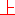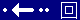Theoretical Law     Last updated on 2014/2558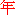10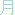8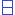, a full moon day;

Associative Law: x * ( y * z ) = ( x * y ) * z

Commutative Law: x * y = y * x

Derivative Property: ( x * y )' = x' * y = x * y'

Distributive Law: x * ( y + z ) = ( x * y ) + ( x * z )     Also see: Distribution; Mathematics;

February 20, 2008, lunar eclipse night, in USA; February 21, 2008, lunar eclipse day, and also a full moon day in Asia ... ; Earth may shake a bit ... ;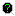ACT2 2 * 7 Lunar Time 2 * 5 Jun Time Associative Law x * ( y * z ) ( x * y ) * z Commutative Law x * y y * x Derivative Property IFF Propagation Time = 1, ( x * y )' = x' * y = x * y' IFF Propagation Time < 1, ... IFF Propagation Time = 0, ... Distributive Law x * ( y + z ) ( x * y ) + ( x * z )

Note: If Laplace transform to do convolution, please read The Handbook of Mechanical Engineering, Staff of Research & Education Association, REA, 2004;

For Monbusho level developers: develop ACT1 mathematical laws and properties to ACT2 stage by 2*7 Lunar Time and 2*5 Jun Time;

...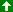Up